# Ordered sum

(diff) ← Older revision | Latest revision (diff) | Newer revision → (diff)
An operation which associates with a system of disjoint partially ordered sets, where the index setis also partially ordered, a new partially ordered set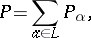the elements of which are the elements of the set-theoretical union of the sets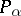, with order defined as follows. On the setone hasif and only if either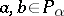and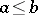inor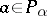,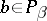and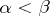. Important particular cases of ordered sums are the cardinal and ordinal sums. The first of these is obtained whenis trivially ordered, i.e. each of its elements is comparable only to itself, and the second whenis a totally ordered set. Thus, in the cardinal sum of two disjoint partially ordered setsandthe relation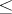retains its meaning in the componentsand, whileand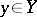are incomparable; in the ordinal sum ofandthe order relation is again preserved in the components and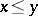for all,.